# TIGRAMITE¶

Github repo

Tigramite is a causal time series analysis python package. It allows to efficiently reconstruct causal graphs from high-dimensional time series datasets and model the obtained causal dependencies for causal mediation and prediction analyses. Causal discovery is based on linear as well as non-parametric conditional independence tests applicable to discrete or continuously-valued time series. Also includes functions for high-quality plots of the results. Please cite the following papers depending on which method you use:

1. J. Runge et al. (2019): Inferring causation from time series in Earth system sciences. Nature Communications, 10(1):2553. https://www.nature.com/articles/s41467-019-10105-3

2. J. Runge, P. Nowack, M. Kretschmer, S. Flaxman, D. Sejdinovic (2019): Detecting and quantifying causal associations in large nonlinear time series datasets. Sci. Adv. 5, eaau4996. https://advances.sciencemag.org/content/5/11/eaau4996

3. J. Runge et al. (2015): Identifying causal gateways and mediators in complex spatio-temporal systems. Nature Communications, 6, 8502. http://doi.org/10.1038/ncomms9502

4. J. Runge (2015): Quantifying information transfer and mediation along causal pathways in complex systems. Phys. Rev. E, 92(6), 62829. http://doi.org/10.1103/PhysRevE.92.062829

5. J. Runge (2018): Conditional Independence Testing Based on a Nearest-Neighbor Estimator of Conditional Mutual Information. In Proceedings of the 21st International Conference on Artificial Intelligence and Statistics. http://proceedings.mlr.press/v84/runge18a.html

6. J. Runge (2018): Causal Network Reconstruction from Time Series: From Theoretical Assumptions to Practical Estimation. Chaos: An Interdisciplinary Journal of Nonlinear Science 28 (7): 075310. https://aip.scitation.org/doi/10.1063/1.5025050

 tigramite.pcmci.PCMCI(dataframe, cond_ind_test) PCMCI causal discovery for time series datasets. Base class of conditional independence tests. Partial correlation test. GPDC conditional independence test based on Gaussian processes and Conditional mutual information test based on nearest-neighbor estimator. Conditional mutual information test based on discrete estimator. tigramite.independence_tests.RCOT([num_f, …]) Randomized Conditional Correlation Test. tigramite.data_processing Tigramite data processing functions. tigramite.models.Models(dataframe, model[, …]) Base class for time series models. tigramite.models.LinearMediation(dataframe) Linear mediation analysis for time series models. tigramite.models.Prediction(dataframe, …) Prediction class for time series models. tigramite.plotting Tigramite plotting package.

# tigramite.pcmci: PCMCI¶

class tigramite.pcmci.PCMCI(dataframe, cond_ind_test, selected_variables=None, verbosity=0)[source]

PCMCI causal discovery for time series datasets.

PCMCI is a 2-step causal discovery method for large-scale time series datasets. The first step is a condition-selection followed by the MCI conditional independence test. The implementation is based on Algorithms 1 and 2 in 1.

PCMCI allows:

• different conditional independence test statistics adapted to continuously-valued or discrete data, and different assumptions about linear or nonlinear dependencies

• hyperparameter optimization

• easy parallelization

• handling of masked time series data

• false discovery control and confidence interval estimation

Notes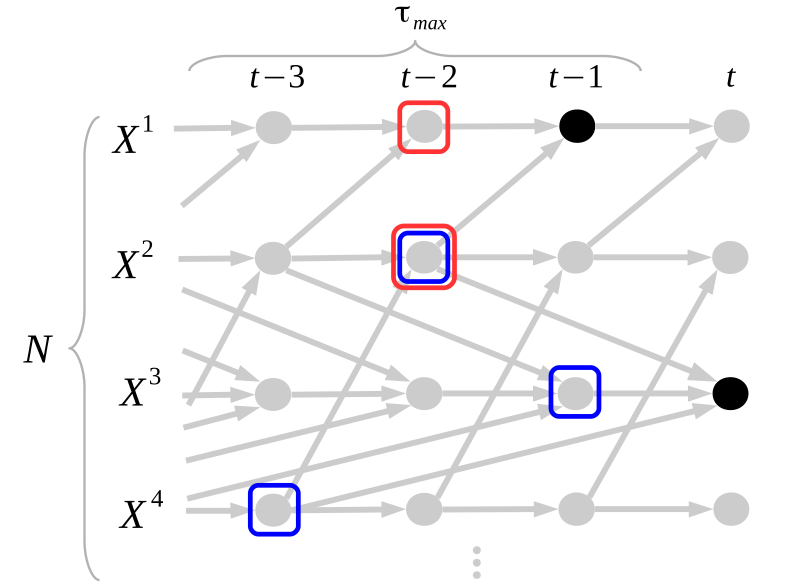The PCMCI causal discovery method is comprehensively described in 1, where also analytical and numerical results are presented. Here we briefly summarize the method.

In the PCMCI framework, the dependency structure of a set of time series variables is represented in a time series graph as shown in the Figure. The nodes of a time series graph are defined as the variables at different times and a link exists if two lagged variables are not conditionally independent given the past of the whole process. Assuming stationarity, the links are repeated in time. The parents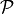of a variable are defined as the set of all nodes with a link towards it (blue and red boxes in Figure). Estimating these parents directly by testing for conditional independence on the whole past is problematic due to high-dimensionality and because conditioning on irrelevant variables leads to biases 1.

PCMCI estimates causal links by a two-step procedure:

1. Condition-selection: For each variable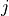, estimate a superset of parentswith the iterative PC1 algorithm , implemented as run_pc_stable.

2. Momentary conditional independence (MCI)here implemented as run_mci. The condition-selection step reduces the dimensionality and avoids conditioning on irrelevant variables.

PCMCI can be flexibly combined with any kind of conditional independence test statistic adapted to the kind of data (continuous or discrete) and its assumed dependency structure. Currently, implemented in Tigramite are ParCorr as a linear test, GPACE allowing nonlinear additive dependencies, and CMI with different estimators making no assumptions about the dependencies. The classes in tigramite.independence_tests also handle masked data.

The main free parameters of PCMCI (in addition to free parameters of the conditional independence test statistic) are the maximum time delay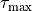(tau_max) and the significance threshold in the condition- selection step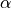(pc_alpha). The maximum time delay depends on the application and should be chosen according to the maximum causal time lag expected in the complex system. We recommend a rather large choice that includes peaks in the lagged cross-correlation function (or a more general measure).should not be seen as a significance test level in the condition-selection step since the iterative hypothesis tests do not allow for a precise confidence level.rather takes the role of a regularization parameter in model-selection techniques. The conditioning setsshould include the true parents and at the same time be small in size to reduce the estimation dimension of the MCI test and improve its power. But including the true parents is typically more important. If a list of values is given or pc_alpha=None,is optimized using model selection criteria.

Further optional parameters are discussed in 1.

References

1(1,2,3,4,5,6,7,8,9,10,11,12,13,14,15,16,17,18,19)

J. Runge, P. Nowack, M. Kretschmer, S. Flaxman, D. Sejdinovic, Detecting and quantifying causal associations in large nonlinear time series datasets. Sci. Adv. 5, eaau4996 (2019) https://advances.sciencemag.org/content/5/11/eaau4996

Examples

>>> import numpy
>>> from tigramite.pcmci import PCMCI
>>> from tigramite.independence_tests import ParCorr
>>> import tigramite.data_processing as pp
>>> numpy.random.seed(42)
>>> # Example process to play around with
>>> # Each key refers to a variable and the incoming links are supplied as a
>>> # list of format [((driver, lag), coeff), ...]
>>> links_coeffs = {0: [((0, -1), 0.8)],
1: [((1, -1), 0.8), ((0, -1), 0.5)],
2: [((2, -1), 0.8), ((1, -2), -0.6)]}
>>> data, _ = pp.var_process(links_coeffs, T=1000)
>>> # Data must be array of shape (time, variables)
>>> print data.shape
(1000, 3)
>>> dataframe = pp.DataFrame(data)
>>> cond_ind_test = ParCorr()
>>> pcmci = PCMCI(dataframe=dataframe, cond_ind_test=cond_ind_test)
>>> results = pcmci.run_pcmci(tau_max=2, pc_alpha=None)
val_matrix=results['val_matrix'],
alpha_level=0.05)
## Significant parents at alpha = 0.05:
Variable 0 has 1 parent(s):
(0 -1): pval = 0.00000 | val = 0.623
Variable 1 has 2 parent(s):
(1 -1): pval = 0.00000 | val = 0.601
(0 -1): pval = 0.00000 | val = 0.487
Variable 2 has 2 parent(s):
(2 -1): pval = 0.00000 | val = 0.597
(1 -2): pval = 0.00000 | val = -0.511

Parameters
• dataframe (data object) – This is the Tigramite dataframe object. It has the attributes dataframe.values yielding a numpy array of shape (observations T, variables N) and optionally a mask of the same shape.

• cond_ind_test (conditional independence test object) –

This can be ParCorr or other classes from the tigramite package or an external test passed as a callable. This test can be based on the class tigramite.independence_tests.CondIndTest. If a callable is passed, it must have the signature:

class CondIndTest():
# with attributes
# * measure : str
#   name of the test
#   whether the mask should be used

# and functions
# * run_test(X, Y, Z, tau_max) : where X,Y,Z are of the form
#   X = [(var, -tau)]  for non-negative integers var and tau
#   specifying the variable and time lag
#   return (test statistic value, p-value)
# * set_dataframe(dataframe) : set dataframe object

# optionally also

# * get_model_selection_criterion(j, parents) : required if
#   pc_alpha parameter is to be optimized. Here j is the
#   variable index and parents a list [(var, -tau), ...]
#   return score for model selection
# * get_confidence(X, Y, Z, tau_max) : required for
#   return_confidence=True
#   estimate confidence interval after run_test was called
#   return (lower bound, upper bound)


• selected_variables (list of integers, optional (default: range(N))) – Specify to estimate parents only for selected variables. If None is passed, parents are estimated for all variables. Note that parents can still come from all variables, you can restrict parents using the selected_links parameter.

• verbosity (int, optional (default: 0)) – Verbose levels 0, 1, …

all_parents

Dictionary of form {0:[(0, -1), (3, -2), …], 1:[], …} containing the conditioning-parents estimated with PC algorithm.

Type

dictionary

val_min

Dictionary of form val_min[j][(i, -tau)] = float containing the minimum test statistic value for each link estimated in the PC algorithm.

Type

dictionary

p_max

Dictionary of form p_max[j][(i, -tau)] = float containing the maximum p-value for each link estimated in the PC algorithm.

Type

dictionary

iterations

Dictionary containing further information on algorithm steps.

Type

dictionary

N

Number of variables.

Type

int

T

Time series sample length.

Type

int

get_corrected_pvalues(p_matrix, fdr_method='fdr_bh', exclude_contemporaneous=True)[source]

Returns p-values corrected for multiple testing.

Currently implemented is Benjamini-Hochberg False Discovery Rate method. Correction is performed either among all links if exclude_contemporaneous==False, or only among lagged links.

Parameters
• p_matrix (array-like) – Matrix of p-values. Must be of shape (N, N, tau_max + 1).

• fdr_method (str, optional (default: 'fdr_bh')) – Correction method, currently implemented is Benjamini-Hochberg False Discovery Rate method.

• exclude_contemporaneous (bool, optional (default: True)) – Whether to include contemporaneous links in correction.

Returns

q_matrix – Matrix of shape (N, N, tau_max + 1) containing corrected p-values.

Return type

array-like

get_lagged_dependencies(selected_links=None, tau_min=0, tau_max=1, parents=None, max_conds_py=None, max_conds_px=None)[source]

Returns matrix of lagged dependence measure values.

Parameters
• selected_links (dict or None) – Dictionary of form {0:[(0, -1), (3, -2), …], 1:[], …} specifying whether only selected links should be tested. If None is passed, all links are tested

• tau_min (int, default: 0) – Minimum time lag.

• tau_max (int, default: 1) – Maximum time lag. Must be larger or equal to tau_min.

• parents (dict or None) – Dictionary of form {0:[(0, -1), (3, -2), …], 1:[], …} specifying the conditions for each variable. If None is passed, no conditions are used.

• max_conds_py (int or None) – Maximum number of conditions from parents of Y to use. If None is passed, this number is unrestricted.

• max_conds_px (int or None) – Maximum number of conditions from parents of X to use. If None is passed, this number is unrestricted.

Returns

val_matrix – The matrix of shape (N, N, tau_max+1) containing the lagged dependencies.

Return type

array

print_results(return_dict, alpha_level=0.05)[source]

Prints significant parents from output of MCI or PCMCI algorithms.

Parameters
• return_dict (dict) –

Dictionary of return values, containing keys
• ’p_matrix’

• ’val_matrix’

• ’conf_matrix’

’q_matrix’ can also be included in keys, but is not necessary.

• alpha_level (float, optional (default: 0.05)) – Significance level.

Prints significant parents.

Parameters
• alpha_level (float, optional (default: 0.05)) – Significance level.

• p_matrix (array-like) – Must be of shape (N, N, tau_max + 1).

• val_matrix (array-like) – Must be of shape (N, N, tau_max + 1).

• q_matrix (array-like, optional (default: None)) – Adjusted p-values. Must be of shape (N, N, tau_max + 1).

• conf_matrix (array-like, optional (default: None)) – Matrix of confidence intervals of shape (N, N, tau_max+1, 2)

return_significant_parents(pq_matrix, val_matrix, alpha_level=0.05, include_lagzero_parents=False)[source]

Returns list of significant parents as well as a boolean matrix.

Significance based on p-matrix, or q-value matrix with corrected p-values.

Parameters
• pq_matrix (array-like) – p-matrix, or q-value matrix with corrected p-values. Must be of shape (N, N, tau_max + 1).

• val_matrix (array-like) – Matrix of test statistic values. Must be of shape (N, N, tau_max + 1).

• alpha_level (float, optional (default: 0.05)) – Significance level.

• include_lagzero_parents (bool (default: False)) – Whether the parents dictionary should also return parents at lag zero. Note that the link_matrix always contains those.

Returns

• all_parents (dict) – Dictionary of form {0:[(0, -1), (3, -2), …], 1:[], …} containing estimated parents.

• link_matrix (array, shape [N, N, tau_max+1]) – Boolean array with True entries for significant links at alpha_level

run_bivci(selected_links=None, tau_min=0, tau_max=1)[source]

BivCI conditional independence tests.

Implements the BivCI test (see 1). Returns the matrices of test statistic values, p-values, and confidence intervals.

Parameters
• selected_links (dict or None) – Dictionary of form {0:all_parents (3, -2), …], 1:[], …} specifying whether only selected links should be tested. If None is passed, all links are tested

• tau_min (int, default: 0) – Minimum time lag to test. Note that zero-lags are undirected.

• tau_max (int, default: 1) – Maximum time lag. Must be larger or equal to tau_min.

Returns

results – {‘val_matrix’:val_matrix, ‘p_matrix’:p_matrix} are always returned and optionally conf_matrix which is of shape [N, N, tau_max+1,2]

Return type

dictionary of arrays of shape [N, N, tau_max+1]

run_fullci(selected_links=None, tau_min=0, tau_max=1)[source]

FullCI conditional independence tests.

Implements the FullCI test (see 1). Returns the matrices of test statistic values, p-values, and confidence intervals.

Parameters
• selected_links (dict or None) – Dictionary of form {0:all_parents (3, -2), …], 1:[], …} specifying whether only selected links should be tested. If None is passed, all links are tested

• tau_min (int, default: 0) – Minimum time lag to test. Note that zero-lags are undirected.

• tau_max (int, default: 1) – Maximum time lag. Must be larger or equal to tau_min.

Returns

results – {‘val_matrix’:val_matrix, ‘p_matrix’:p_matrix} are always returned and optionally conf_matrix which is of shape [N, N, tau_max+1,2]

Return type

dictionary of arrays of shape [N, N, tau_max+1]

run_mci(selected_links=None, tau_min=0, tau_max=1, parents=None, max_conds_py=None, max_conds_px=None)[source]

MCI conditional independence tests.

Implements the MCI test (Algorithm 2 in 1). Returns the matrices of test statistic values, p-values, and confidence intervals.

Parameters
• selected_links (dict or None) – Dictionary of form {0:all_parents (3, -2), …], 1:[], …} specifying whether only selected links should be tested. If None is passed, all links are tested

• tau_min (int, default: 0) – Minimum time lag to test. Note that zero-lags are undirected.

• tau_max (int, default: 1) – Maximum time lag. Must be larger or equal to tau_min.

• parents (dict or None) – Dictionary of form {0:[(0, -1), (3, -2), …], 1:[], …} specifying the conditions for each variable. If None is passed, no conditions are used.

• max_conds_py (int or None) – Maximum number of conditions of Y to use. If None is passed, this number is unrestricted.

• max_conds_px (int or None) – Maximum number of conditions of Z to use. If None is passed, this number is unrestricted.

Returns

results – {‘val_matrix’:val_matrix, ‘p_matrix’:p_matrix} are always returned and optionally conf_matrix which is of shape [N, N, tau_max+1,2]

Return type

dictionary of arrays of shape [N, N, tau_max+1]

run_pc_stable(selected_links=None, tau_min=1, tau_max=1, save_iterations=False, pc_alpha=0.2, max_conds_dim=None, max_combinations=1)[source]

PC algorithm for estimating parents of all variables.

Parents are made available as self.all_parents

Parameters
• selected_links (dict or None) – Dictionary of form {0:[(0, -1), (3, -2), …], 1:[], …} specifying whether only selected links should be tested. If None is passed, all links are tested

• tau_min (int, default: 1) – Minimum time lag to test. Useful for multi-step ahead predictions. Must be greater zero.

• tau_max (int, default: 1) – Maximum time lag. Must be larger or equal to tau_min.

• save_iterations (bool, default: False) – Whether to save iteration step results such as conditions used.

• pc_alpha (float or list of floats, default: [0.05, 0.1, 0.2, 0.3, 0.4, 0.5]) – Significance level in algorithm. If a list or None is passed, the pc_alpha level is optimized for every variable across the given pc_alpha values using the score computed in cond_ind_test.get_model_selection_criterion()

• max_conds_dim (int or None) – Maximum number of conditions to test. If None is passed, this number is unrestricted.

• max_combinations (int, default: 1) – Maximum number of combinations of conditions of current cardinality to test. Defaults to 1 for PC_1 algorithm. For original PC algorithm a larger number, such as 10, can be used.

Returns

all_parents – Dictionary of form {0:[(0, -1), (3, -2), …], 1:[], …} containing estimated parents.

Return type

dict

run_pcmci(selected_links=None, tau_min=0, tau_max=1, save_iterations=False, pc_alpha=0.05, max_conds_dim=None, max_combinations=1, max_conds_py=None, max_conds_px=None, fdr_method='none')[source]

Run full PCMCI causal discovery for time series datasets.

Wrapper around PC-algorithm function and MCI function.

Parameters
• selected_links (dict or None) – Dictionary of form {0:all_parents (3, -2), …], 1:[], …} specifying whether only selected links should be tested. If None is passed, all links are tested

• tau_min (int, optional (default: 0)) – Minimum time lag to test. Note that zero-lags are undirected.

• tau_max (int, optional (default: 1)) – Maximum time lag. Must be larger or equal to tau_min.

• save_iterations (bool, optional (default: False)) – Whether to save iteration step results such as conditions used.

• pc_alpha (float, optional (default: 0.05)) – Significance level in algorithm.

• max_conds_dim (int, optional (default: None)) – Maximum number of conditions to test. If None is passed, this number is unrestricted.

• max_combinations (int, optional (default: 1)) – Maximum number of combinations of conditions of current cardinality to test. Defaults to 1 for PC_1 algorithm. For original PC algorithm a larger number, such as 10, can be used.

• max_conds_py (int, optional (default: None)) – Maximum number of conditions of Y to use. If None is passed, this number is unrestricted.

• max_conds_px (int, optional (default: None)) – Maximum number of conditions of Z to use. If None is passed, this number is unrestricted.

• fdr_method (str, optional (default: 'none')) – Correction method, default is Benjamini-Hochberg False Discovery Rate method.

Returns

results – {‘val_matrix’:val_matrix, ‘p_matrix’:p_matrix} are always returned and optionally q_matrix and conf_matrix which is of shape [N, N, tau_max+1,2]

Return type

dictionary of arrays of shape [N, N, tau_max+1]

# tigramite.independence_tests: Conditional independence tests¶

Base class:

class tigramite.independence_tests.CondIndTest(mask_type=None, significance='analytic', fixed_thres=0.1, sig_samples=1000, sig_blocklength=None, confidence=None, conf_lev=0.9, conf_samples=100, conf_blocklength=None, recycle_residuals=False, verbosity=0)[source]

Base class of conditional independence tests.

Provides useful general functions for different independence tests such as shuffle significance testing and bootstrap confidence estimation. Also handles masked samples. Other test classes can inherit from this class.

Parameters
• mask_type (str, optional (default = None)) – Must be in {‘y’,’x’,’z’,’xy’,’xz’,’yz’,’xyz’} Masking mode: Indicators for which variables in the dependence measure I(X; Y | Z) the samples should be masked. If None, ‘y’ is used, which excludes all time slices containing masked samples in Y. Explained in 1.

• significance (str, optional (default: 'analytic')) – Type of significance test to use. In this package ‘analytic’, ‘fixed_thres’ and ‘shuffle_test’ are available.

• fixed_thres (float, optional (default: 0.1)) – If significance is ‘fixed_thres’, this specifies the threshold for the absolute value of the dependence measure.

• sig_samples (int, optional (default: 1000)) – Number of samples for shuffle significance test.

• sig_blocklength (int, optional (default: None)) – Block length for block-shuffle significance test. If None, the block length is determined from the decay of the autocovariance as explained in 1.

• confidence (str, optional (default: None)) – Specify type of confidence estimation. If False, numpy.nan is returned. ‘bootstrap’ can be used with any test, for ParCorr also ‘analytic’ is implemented.

• conf_lev (float, optional (default: 0.9)) – Two-sided confidence interval.

• conf_samples (int, optional (default: 100)) – Number of samples for bootstrap.

• conf_blocklength (int, optional (default: None)) – Block length for block-bootstrap. If None, the block length is determined from the decay of the autocovariance as explained in 1.

• recycle_residuals (bool, optional (default: False)) – Specifies whether residuals should be stored. This may be faster, but can cost considerable memory.

• verbosity (int, optional (default: 0)) – Level of verbosity.

get_analytic_confidence(value, df, conf_lev)[source]

Base class assumption that this is not implemented. Concrete classes should override when possible.

get_analytic_significance(value, T, dim)[source]

Base class assumption that this is not implemented. Concrete classes should override when possible.

get_bootstrap_confidence(array, xyz, dependence_measure=None, conf_samples=100, conf_blocklength=None, conf_lev=0.95, verbosity=0)[source]

Perform bootstrap confidence interval estimation.

With conf_blocklength > 1 or None a block-bootstrap is performed.

Parameters
• array (array-like) – data array with X, Y, Z in rows and observations in columns

• xyz (array of ints) – XYZ identifier array of shape (dim,).

• dependence_measure (function (default = self.get_dependence_measure)) – Dependence measure function must be of form dependence_measure(array, xyz) and return a numeric value

• conf_lev (float, optional (default: 0.9)) – Two-sided confidence interval.

• conf_samples (int, optional (default: 100)) – Number of samples for bootstrap.

• conf_blocklength (int, optional (default: None)) – Block length for block-bootstrap. If None, the block length is determined from the decay of the autocovariance as explained in 1.

• verbosity (int, optional (default: 0)) – Levelof verbosity.

Returns

(conf_lower, conf_upper) – Upper and lower confidence bound of confidence interval.

Return type

Tuple of floats

get_confidence(X, Y, Z=None, tau_max=0)[source]

Perform confidence interval estimation.

Calls the dependence measure and confidence test functions. The child classes can specify a function get_dependence_measure and get_analytic_confidence or get_bootstrap_confidence. If confidence is False, (numpy.nan, numpy.nan) is returned.

Parameters
• Y, Z (X,) – X,Y,Z are of the form [(var, -tau)], where var specifies the variable index and tau the time lag.

• tau_max (int, optional (default: 0)) – Maximum time lag. This may be used to make sure that estimates for different lags in X, Z, all have the same sample size.

Returns

(conf_lower, conf_upper) – Upper and lower confidence bound of confidence interval.

Return type

Tuple of floats

abstract get_dependence_measure(array, xyz)[source]

Abstract function that all concrete classes must instantiate.

get_fixed_thres_significance(value, fixed_thres)[source]

Returns signficance for thresholding test.

Returns 0 if numpy.abs(value) is smaller than fixed_thres and 1 else.

Parameters
• value (number) – Value of test statistic for unshuffled estimate.

• fixed_thres (number) – Fixed threshold, is made positive.

Returns

pval – Returns 0 if numpy.abs(value) is smaller than fixed_thres and 1 else.

Return type

bool

get_measure(X, Y, Z=None, tau_max=0)[source]

Estimate dependence measure.

Calls the dependence measure function. The child classes must specify a function get_dependence_measure.

Parameters
• Y [, Z] (X,) – X,Y,Z are of the form [(var, -tau)], where var specifies the variable index and tau the time lag.

• tau_max (int, optional (default: 0)) – Maximum time lag. This may be used to make sure that estimates for different lags in X, Z, all have the same sample size.

Returns

val – The test statistic value.

Return type

float

get_model_selection_criterion(j, parents, tau_max=0)[source]

Base class assumption that this is not implemented. Concrete classes should override when possible.

get_shuffle_significance(array, xyz, value, return_null_dist=False)[source]

Base class assumption that this is not implemented. Concrete classes should override when possible.

get_significance(val, array, xyz, T, dim, sig_override=None)[source]

Returns the p-value from whichever significance function is specified for this test. If an override is used, then it will call a different function then specified by self.significance

Parameters
• val (float) – Test statistic value.

• array (array-like) – data array with X, Y, Z in rows and observations in columns

• xyz (array of ints) – XYZ identifier array of shape (dim,).

• T (int) – Sample length

• dim (int) – Dimensionality, ie, number of features.

• sig_override (string) – Must be in ‘analytic’, ‘shuffle_test’, ‘fixed_thres’

Returns

pval – P-value.

Return type

float or numpy.nan

abstract property measure

Abstract property to store the type of independence test.

print_info()[source]

Print information about the conditional independence test parameters

run_test(X, Y, Z=None, tau_max=0, cut_off='2xtau_max')[source]

Perform conditional independence test.

Calls the dependence measure and signficicance test functions. The child classes must specify a function get_dependence_measure and either or both functions get_analytic_significance and get_shuffle_significance. If recycle_residuals is True, also _get_single_residuals must be available.

Parameters
• Y, Z (X,) – X,Y,Z are of the form [(var, -tau)], where var specifies the variable index and tau the time lag.

• tau_max (int, optional (default: 0)) – Maximum time lag. This may be used to make sure that estimates for different lags in X, Z, all have the same sample size.

• cut_off ({'2xtau_max', 'max_lag', 'max_lag_or_tau_max'}) – How many samples to cutoff at the beginning. The default is ‘2xtau_max’, which guarantees that MCI tests are all conducted on the same samples. For modeling, ‘max_lag_or_tau_max’ can be used, which uses the maximum of tau_max and the conditions, which is useful to compare multiple models on the same sample. Last, ‘max_lag’ uses as much samples as possible.

Returns

val, pval – The test statistic value and the p-value.

Return type

Tuple of floats

run_test_raw(x, y, z=None)[source]

Perform conditional independence test directly on input arrays x, y, z.

Calls the dependence measure and signficicance test functions. The child classes must specify a function get_dependence_measure and either or both functions get_analytic_significance and get_shuffle_significance.

Parameters

y, z (x,) – x,y,z are of the form (samples, dimension).

Returns

val, pval – The test statistic value and the p-value.

Return type

Tuple of floats

set_dataframe(dataframe)[source]

Initialize and check the dataframe.

Parameters

dataframe (data object) – Set tigramite dataframe object. It must have the attributes dataframe.values yielding a numpy array of shape (observations T, variables N) and optionally a mask of the same shape and a missing values flag.

set_mask_type(mask_type)[source]

Setter for mask type to ensure that this option does not clash with recycle_residuals.

Parameters

mask_type (str) – Must be in {‘y’,’x’,’z’,’xy’,’xz’,’yz’,’xyz’} Masking mode: Indicators for which variables in the dependence measure I(X; Y | Z) the samples should be masked. If None, ‘y’ is used, which excludes all time slices containing masked samples in Y. Explained in 1.

Test statistics:

class tigramite.independence_tests.ParCorr(**kwargs)[source]

Partial correlation test.

Partial correlation is estimated through linear ordinary least squares (OLS) regression and a test for non-zero linear Pearson correlation on the residuals.

Notes

To test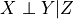, first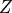is regressed out from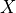and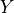assuming the model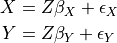using OLS regression. Then the dependency of the residuals is tested with the Pearson correlation test.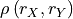For the significance='analytic' Student’s-t distribution with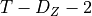degrees of freedom is implemented.

Parameters

**kwargs – Arguments passed on to Parent class CondIndTest.

get_analytic_confidence(value, df, conf_lev)[source]

Returns analytic confidence interval for correlation coefficient.

Based on Student’s t-distribution.

Parameters
• value (float) – Test statistic value.

• df (int) – degrees of freedom of the test

• conf_lev (float) – Confidence interval, eg, 0.9

Returns

(conf_lower, conf_upper) – Upper and lower confidence bound of confidence interval.

Return type

Tuple of floats

get_analytic_significance(value, T, dim)[source]

Returns analytic p-value from Student’s t-test for the Pearson correlation coefficient.

Assumes two-sided correlation. If the degrees of freedom are less than 1, numpy.nan is returned.

Parameters
• value (float) – Test statistic value.

• T (int) – Sample length

• dim (int) – Dimensionality, ie, number of features.

Returns

pval – P-value.

Return type

float or numpy.nan

get_dependence_measure(array, xyz)[source]

Return partial correlation.

Estimated as the Pearson correlation of the residuals of a linear OLS regression.

Parameters
• array (array-like) – data array with X, Y, Z in rows and observations in columns

• xyz (array of ints) – XYZ identifier array of shape (dim,).

Returns

val – Partial correlation coefficient.

Return type

float

get_model_selection_criterion(j, parents, tau_max=0)[source]

Returns Akaike’s Information criterion modulo constants.

Fits a linear model of the parents to variable j and returns the score. I used to determine optimal hyperparameters in PCMCI, in particular the pc_alpha value.

Parameters
• j (int) – Index of target variable in data array.

• parents (list) – List of form [(0, -1), (3, -2), …] containing parents.

• tau_max (int, optional (default: 0)) – Maximum time lag. This may be used to make sure that estimates for different lags in X, Z, all have the same sample size.

• Returns

• score (float) – Model score.

get_shuffle_significance(array, xyz, value, return_null_dist=False)[source]

Returns p-value for shuffle significance test.

For residual-based test statistics only the residuals are shuffled.

Parameters
• array (array-like) – data array with X, Y, Z in rows and observations in columns

• xyz (array of ints) – XYZ identifier array of shape (dim,).

• value (number) – Value of test statistic for unshuffled estimate.

Returns

pval – p-value

Return type

float

property measure

Concrete property to return the measure of the independence test

class tigramite.independence_tests.GPDC(null_dist_filename=None, gp_version='new', gp_params=None, **kwargs)[source]
GPDC conditional independence test based on Gaussian processes and

distance correlation.

GPDC is based on a Gaussian process (GP) regression and a distance correlation test on the residuals 2. GP is estimated with scikit-learn and allows to flexibly specify kernels and hyperparameters or let them be optimized automatically. The distance correlation test is implemented with cython. Here the null distribution is not analytically available, but can be precomputed with the function generate_and_save_nulldists(…) which saves a *.npz file containing the null distribution for different sample sizes. This file can then be supplied as null_dist_filename.

Notes

GPDC is based on a Gaussian process (GP) regression and a distance correlation test on the residuals. Distance correlation is described in 2. To test, firstis regressed out fromandassuming the model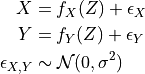using GP regression. Here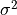and the kernel bandwidth are optimzed using sklearn. Then the residuals are transformed to uniform marginals yielding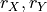and their dependency is tested with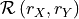The null distribution of the distance correlation should be pre-computed. Otherwise it is computed during runtime.

The cython-code for distance correlation is Copyright (c) 2012, Florian Finkernagel (https://gist.github.com/ffinkernagel/2960386).

References

2(1,2)

Gabor J. Szekely, Maria L. Rizzo, and Nail K. Bakirov: Measuring and testing dependence by correlation of distances, https://arxiv.org/abs/0803.4101

Parameters
• null_dist_filename (str, otional (default: None)) – Path to file containing null distribution.

• gp_version ({'new', 'old'}, optional (default: 'new')) – The older GP version from scikit-learn 0.17 was used for the numerical simulations in 1. The newer version from scikit-learn 0.19 is faster and allows more flexibility regarding kernels etc.

• gp_params (dictionary, optional (default: None)) – Dictionary with parameters for GaussianProcessRegressor.

• **kwargs – Arguments passed on to parent class GaussProcReg.

generate_and_save_nulldists(sample_sizes, null_dist_filename)[source]

Generates and saves null distribution for pairwise independence tests.

Generates the null distribution for different sample sizes. Calls generate_nulldist. Null dists are saved to disk as self.null_dist_filename.npz. Also adds the null distributions to self.gauss_pr.null_dists.

Parameters
• sample_sizes (list) – List of sample sizes.

• null_dist_filename (str) – Name to save file containing null distributions.

generate_nulldist(df, add_to_null_dists=True)[source]

Generates null distribution for pairwise independence tests.

Generates the null distribution for sample size df. Assumes pairwise samples transformed to uniform marginals. Uses get_dependence_measure available in class and generates self.sig_samples random samples. Adds the null distributions to self.gauss_pr.null_dists.

Parameters
• df (int) – Degrees of freedom / sample size to generate null distribution for.

• add_to_null_dists (bool, optional (default: True)) – Whether to add the null dist to the dictionary of null dists or just return it.

Returns

null_dist – Only returned,if add_to_null_dists is False.

Return type

array of shape [df,]

get_analytic_significance(value, T, dim)[source]

Returns p-value for the distance correlation coefficient.

The null distribution for necessary degrees of freedom (df) is loaded. If not available, the null distribution is generated with the function generate_nulldist(). It is recommended to generate the nulldists for a wide range of sample sizes beforehand with the function generate_and_save_nulldists(…). The distance correlation coefficient is one-sided. If the degrees of freedom are less than 1, numpy.nan is returned.

Parameters
• value (float) – Test statistic value.

• T (int) – Sample length

• dim (int) – Dimensionality, ie, number of features.

Returns

pval – P-value.

Return type

float or numpy.nan

get_dependence_measure(array, xyz)[source]

Return GPDC measure.

Estimated as the distance correlation of the residuals of a GP regression.

Parameters
• array (array-like) – data array with X, Y, Z in rows and observations in columns

• xyz (array of ints) – XYZ identifier array of shape (dim,).

Returns

val – GPDC test statistic.

Return type

float

get_model_selection_criterion(j, parents, tau_max=0)[source]

Returns log marginal likelihood for GP regression.

Fits a GP model of the parents to variable j and returns the negative log marginal likelihood as a model selection score. Is used to determine optimal hyperparameters in PCMCI, in particular the pc_alpha value.

Parameters
• j (int) – Index of target variable in data array.

• parents (list) – List of form [(0, -1), (3, -2), …] containing parents.

• tau_max (int, optional (default: 0)) – Maximum time lag. This may be used to make sure that estimates for different lags in X, Z, all have the same sample size.

• Returns

• score (float) – Model score.

get_shuffle_significance(array, xyz, value, return_null_dist=False)[source]

Returns p-value for shuffle significance test.

For residual-based test statistics only the residuals are shuffled.

Parameters
• array (array-like) – data array with X, Y, Z in rows and observations in columns

• xyz (array of ints) – XYZ identifier array of shape (dim,).

• value (number) – Value of test statistic for unshuffled estimate.

Returns

pval – p-value

Return type

float

property measure

Concrete property to return the measure of the independence test

class tigramite.independence_tests.CMIknn(knn=0.2, shuffle_neighbors=5, significance='shuffle_test', transform='ranks', n_jobs=-1, **kwargs)[source]

Conditional mutual information test based on nearest-neighbor estimator.

Conditional mutual information is the most general dependency measure coming from an information-theoretic framework. It makes no assumptions about the parametric form of the dependencies by directly estimating the underlying joint density. The test here is based on the estimator in S. Frenzel and B. Pompe, Phys. Rev. Lett. 99, 204101 (2007), combined with a shuffle test to generate the distribution under the null hypothesis of independence first used in 3. The knn-estimator is suitable only for variables taking a continuous range of values. For discrete variables use the CMIsymb class.

Notes

CMI is given by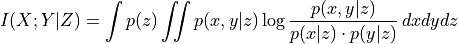Its knn-estimator is given by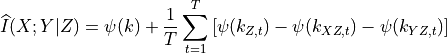where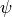is the Digamma function. This estimator has as a parameter the number of nearest-neighbors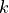which determines the size of hyper-cubes around each (high-dimensional) sample point. Then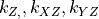are the numbers of neighbors in the respective subspaces.can be viewed as a density smoothing parameter (although it is data-adaptive unlike fixed-bandwidth estimators). For large, the underlying dependencies are more smoothed and CMI has a larger bias, but lower variance, which is more important for significance testing. Note that the estimated CMI values can be slightly negative while CMI is a non- negative quantity.

This method requires the scipy.spatial.cKDTree package and the tigramite cython module.

References

3

J. Runge (2018): Conditional Independence Testing Based on a Nearest-Neighbor Estimator of Conditional Mutual Information. In Proceedings of the 21st International Conference on Artificial Intelligence and Statistics. http://proceedings.mlr.press/v84/runge18a.html

Parameters
• knn (int or float, optional (default: 0.2)) – Number of nearest-neighbors which determines the size of hyper-cubes around each (high-dimensional) sample point. If smaller than 1, this is computed as a fraction of T, hence knn=knn*T. For knn larger or equal to 1, this is the absolute number.

• shuffle_neighbors (int, optional (default: 10)) – Number of nearest-neighbors within Z for the shuffle surrogates which determines the size of hyper-cubes around each (high-dimensional) sample point.

• transform ({'ranks', 'standardize', 'uniform', False}, optional) – (default: ‘ranks’) Whether to transform the array beforehand by standardizing or transforming to uniform marginals.

• n_jobs (int (optional, default = -1)) – Number of jobs to schedule for parallel processing. If -1 is given all processors are used. Default: 1.

• significance (str, optional (default: 'shuffle_test')) – Type of significance test to use. For CMIknn only ‘fixed_thres’ and ‘shuffle_test’ are available.

• **kwargs – Arguments passed on to parent class CondIndTest.

get_dependence_measure(array, xyz)[source]

Returns CMI estimate as described in Frenzel and Pompe PRL (2007).

Parameters
• array (array-like) – data array with X, Y, Z in rows and observations in columns

• xyz (array of ints) – XYZ identifier array of shape (dim,).

Returns

val – Conditional mutual information estimate.

Return type

float

get_shuffle_significance(array, xyz, value, return_null_dist=False)[source]

Returns p-value for nearest-neighbor shuffle significance test.

For non-empty Z, overwrites get_shuffle_significance from the parent class which is a block shuffle test, which does not preserve dependencies of X and Y with Z. Here the parameter shuffle_neighbors is used to permute only those values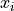and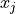for which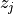is among the nearest niehgbors of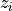. If Z is empty, the block-shuffle test is used.

Parameters
• array (array-like) – data array with X, Y, Z in rows and observations in columns

• xyz (array of ints) – XYZ identifier array of shape (dim,).

• value (number) – Value of test statistic for unshuffled estimate.

Returns

pval – p-value

Return type

float

property measure

Concrete property to return the measure of the independence test

class tigramite.independence_tests.CMIsymb(n_symbs=None, significance='shuffle_test', sig_blocklength=1, conf_blocklength=1, **kwargs)[source]

Conditional mutual information test based on discrete estimator.

Conditional mutual information is the most general dependency measure coming from an information-theoretic framework. It makes no assumptions about the parametric form of the dependencies by directly estimating the underlying joint density. The test here is based on directly estimating the joint distribution assuming symbolic input, combined with a shuffle test to generate the distribution under the null hypothesis of independence. The knn-estimator is suitable only for discrete variables. For continuous variables, either pre-process the data using the functions in data_processing or, better, use the CMIknn class.

Notes

CMI and its estimator are given by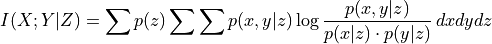Parameters
• n_symbs (int, optional (default: None)) – Number of symbols in input data. Should be at least as large as the maximum array entry + 1. If None, n_symbs is based on the maximum value in the array (array.max() + 1).

• significance (str, optional (default: 'shuffle_test')) – Type of significance test to use. For CMIsymb only ‘fixed_thres’ and ‘shuffle_test’ are available.

• sig_blocklength (int, optional (default: 1)) – Block length for block-shuffle significance test.

• conf_blocklength (int, optional (default: 1)) – Block length for block-bootstrap.

• **kwargs – Arguments passed on to parent class CondIndTest.

get_dependence_measure(array, xyz)[source]

Returns CMI estimate based on bincount histogram.

Parameters
• array (array-like) – data array with X, Y, Z in rows and observations in columns

• xyz (array of ints) – XYZ identifier array of shape (dim,).

Returns

val – Conditional mutual information estimate.

Return type

float

get_shuffle_significance(array, xyz, value, return_null_dist=False)[source]

Returns p-value for shuffle significance test.

For residual-based test statistics only the residuals are shuffled.

Parameters
• array (array-like) – data array with X, Y, Z in rows and observations in columns

• xyz (array of ints) – XYZ identifier array of shape (dim,).

• value (number) – Value of test statistic for unshuffled estimate.

Returns

pval – p-value

Return type

float

property measure

Concrete property to return the measure of the independence test

class tigramite.independence_tests.RCOT(num_f=25, approx='lpd4', seed=42, significance='analytic', **kwargs)[source]

Randomized Conditional Correlation Test.

Tests conditional independence in the fully non-parametric setting based on Kernel measures. For not too small sample sizes, the test can utilize an analytic approximation of the null distribution making it very fast. Based on r-package rcit. This test is described in 5.

Notes

RCOT is a fast variant of the Kernel Conditional Independence Test (KCIT) utilizing random Fourier features. Kernel tests measure conditional independence in the fully non-parametric setting. In practice, RCOT tests scale linearly with sample size and return accurate p-values much faster than KCIT in the large sample size context. To use the analytical null approximation, the sample size should be at least ~1000.

The method is fully described in 5 and the r-package documentation. The free parameters are the approximation of the partial kernel cross-covariance matrix and the number of random fourier features for the conditioning set. One caveat is that RCOT is, as the name suggests, based on random fourier features. To get reproducable results, you should fix the seed (default).

This class requires the rpy package and the prior installation of rcit from https://github.com/ericstrobl/RCIT. This is provided with tigramite as an external package.

References

5(1,2)

Eric V. Strobl, Kun Zhang, Shyam Visweswaran: Approximate Kernel-based Conditional Independence Tests for Fast Non- Parametric Causal Discovery. https://arxiv.org/abs/1702.03877

Parameters
• num_f (int, optional) – Number of random fourier features for conditioning set. More features better approximate highly structured joint densities, but take more computational time.

• approx (str, optional) – Which approximation of the partial cross-covariance matrix, options: ‘lpd4’ the Lindsay-Pilla-Basak method (default), ‘gamma’ for the Satterthwaite-Welch method, ‘hbe’ for the Hall-Buckley-Eagleson method, ‘chi2’ for a normalized chi-squared statistic, ‘perm’ for permutation testing (warning: this one is slow).

• seed (int or None, optional) – Which random fourier feature seed to use. If None, you won’t get reproducable results.

• significance (str, optional (default: 'analytic')) – Type of significance test to use.

• **kwargs – Arguments passed on to parent class CondIndTest.

get_analytic_significance(**args)[source]

Returns analytic p-value from RCoT test statistic. NOTE: Must first run get_dependence_measure, where p-value is determined from RCoT test statistic.

Returns

pval – P-value.

Return type

float or numpy.nan

get_dependence_measure(array, xyz)[source]

Returns RCOT estimate.

Parameters
• array (array-like) – data array with X, Y, Z in rows and observations in columns

• xyz (array of ints) – XYZ identifier array of shape (dim,).

Returns

val – RCOT estimate.

Return type

float

get_shuffle_significance(array, xyz, value, return_null_dist=False)[source]

Returns p-value for shuffle significance test.

For residual-based test statistics only the residuals are shuffled.

Parameters
• array (array-like) – data array with X, Y, Z in rows and observations in columns

• xyz (array of ints) – XYZ identifier array of shape (dim,).

• value (number) – Value of test statistic for unshuffled estimate.

Returns

pval – p-value

Return type

float

property measure

Concrete property to return the measure of the independence test

# tigramite.data_processing: Data processing functions¶

Tigramite data processing functions.

class tigramite.data_processing.DataFrame(data, mask=None, missing_flag=None, var_names=None, datatime=None)[source]

Data object containing time series array and optional mask.

Alternatively, a panda dataframe can be used.

Parameters
• data (array-like) – Numpy array of shape (observations T, variables N)

• mask (array-like, optional (default: None)) – Optional mask array, must be of same shape as data

data

Numpy array of shape (observations T, variables N)

Type

array-like

mask

Optional mask array, must be of same shape as data

Type

array-like, optional (default: None)

missing_flag

Flag for missing values in dataframe. Dismisses all time slices of samples where missing values occur in any variable and also flags samples for all lags up to 2*tau_max. This avoids biases, see section on masking in Supplement of 1.

Type

number, optional (default: None)

var_names

Names of variables, must match the number of variables. If None is passed, variables are enumerated as [0, 1, …]

Type

list of strings, optional (default: range(N))

datatime

Timelabel array. If None, range(T) is used.

Type

array-like, optional (default: None)

construct_array(X, Y, Z, tau_max, mask=None, mask_type=None, return_cleaned_xyz=False, do_checks=True, cut_off='2xtau_max', verbosity=0)[source]

Constructs array from variables X, Y, Z from data.

Data is of shape (T, N), where T is the time series length and N the number of variables.

Parameters
• Y, Z (X,) – For a dependence measure I(X;Y|Z), Y is of the form [(varY, 0)], where var specifies the variable index. X typically is of the form [(varX, -tau)] with tau denoting the time lag and Z can be multivariate [(var1, -lag), (var2, -lag), …] .

• tau_max (int) – Maximum time lag. This may be used to make sure that estimates for different lags in X and Z all have the same sample size.

• mask (array-like, optional (default: None)) – Optional mask array, must be of same shape as data. If it is set, then it overrides the self.mask assigned to the dataframe. If it is None, then the self.mask is used, if it exists.

• mask_type ({'y','x','z','xy','xz','yz','xyz'}) – Masking mode: Indicators for which variables in the dependence measure I(X; Y | Z) the samples should be masked. If None, ‘y’ is used, which excludes all time slices containing masked samples in Y. Explained in 1.

• return_cleaned_xyz (bool, optional (default: False)) – Whether to return cleaned X,Y,Z, where possible duplicates are removed.

• do_checks (bool, optional (default: True)) – Whether to perform sanity checks on input X,Y,Z

• cut_off ({'2xtau_max', 'max_lag', 'max_lag_or_tau_max'}) – How many samples to cutoff at the beginning. The default is ‘2xtau_max’, which guarantees that MCI tests are all conducted on the same samples. For modeling, ‘max_lag_or_tau_max’ can be used, which uses the maximum of tau_max and the conditions, which is useful to compare multiple models on the same sample. Last, ‘max_lag’ uses as much samples as possible.

• verbosity (int, optional (default: 0)) – Level of verbosity.

Returns

array, xyz [,XYZ] – identifier array of shape (dim,) identifying which row in array corresponds to X, Y, and Z. For example:

X = [(0, -1)], Y = [(1, 0)], Z = [(1, -1), (0, -2)]
yields an array of shape (5, T) and xyz is
xyz = numpy.array([0,1,2,2])


If return_cleaned_xyz is True, also outputs the cleaned XYZ lists.

Return type

Tuple of data array of shape (dim, T) and xyz

print_array_info(array, X, Y, Z, missing_flag, mask_type)[source]

Print info about the constructed array

Parameters
• array (Data array of shape (dim, T)) –

• Y, Z (X,) – For a dependence measure I(X;Y|Z), Y is of the form [(varY, 0)], where var specifies the variable index. X typically is of the form [(varX, -tau)] with tau denoting the time lag and Z can be multivariate [(var1, -lag), (var2, -lag), …] .

• missing_flag (number, optional (default: None)) – Flag for missing values. Dismisses all time slices of samples where missing values occur in any variable and also flags samples for all lags up to 2*tau_max. This avoids biases, see section on masking in Supplement of 1.

• mask_type ({'y','x','z','xy','xz','yz','xyz'}) – Masking mode: Indicators for which variables in the dependence measure I(X; Y | Z) the samples should be masked. If None, ‘y’ is used, which excludes all time slices containing masked samples in Y. Explained in 1.

tigramite.data_processing.lowhighpass_filter(data, cutperiod, pass_periods='low')[source]

Butterworth low- or high pass filter.

This function applies a linear filter twice, once forward and once backwards. The combined filter has linear phase.

Parameters
• data (array) – Data array of shape (time, variables).

• cutperiod (int) – Period of cutoff.

• pass_periods (str, optional (default: 'low')) – Either ‘low’ or ‘high’ to act as a low- or high-pass filter

Returns

data – Filtered data array.

Return type

array

tigramite.data_processing.ordinal_patt_array(array, array_mask=None, dim=2, step=1, weights=False, verbosity=0)[source]

Returns symbolified array of ordinal patterns.

Each data vector (X_t, …, X_t+(dim-1)*step) is converted to its rank vector. E.g., (0.2, -.6, 1.2) –> (1,0,2) which is then assigned to a unique integer (see Article). There are faculty(dim) possible rank vectors.

Note that the symb_array is step*(dim-1) shorter than the original array!

Reference: B. Pompe and J. Runge (2011). Momentary information transfer as a coupling measure of time series. Phys. Rev. E, 83(5), 1-12. doi:10.1103/PhysRevE.83.051122

Parameters
• array (array-like) – Data array of shape (time, variables).

• dim (int, optional (default: 2)) – Pattern dimension

• step (int, optional (default: 1)) – Delay of pattern embedding vector.

• weights (bool, optional (default: False)) – Whether to return array of variances of embedding vectors as weights.

• verbosity (int, optional (default: 0)) – Level of verbosity.

Returns

patt, patt_mask [, patt_time] – Tuple of converted pattern array and new length

Return type

tuple of arrays

tigramite.data_processing.quantile_bin_array(data, bins=6)[source]

Returns symbolified array with equal-quantile binning.

Parameters
• data (array) – Data array of shape (time, variables).

• bins (int, optional (default: 6)) – Number of bins.

Returns

symb_array – Converted data of integer type.

Return type

array

tigramite.data_processing.smooth(data, smooth_width, kernel='gaussian', mask=None, residuals=False)[source]

Returns either smoothed time series or its residuals.

the difference between the original and the smoothed time series (=residuals) of a kernel smoothing with gaussian (smoothing kernel width = twice the sigma!) or heaviside window, equivalent to a running mean.

Assumes data of shape (T, N) or (T,) :rtype: array :returns: smoothed/residual data

Parameters
• data (array) – Data array of shape (time, variables).

• smooth_width (float) – Window width of smoothing, 2*sigma for a gaussian.

• kernel (str, optional (default: 'gaussian')) – Smoothing kernel, ‘gaussian’ or ‘heaviside’ for a running mean.

• residuals (bool, optional (default: False)) – True if residuals should be returned instead of smoothed data.

Returns

data – Smoothed/residual data.

Return type

array-like

tigramite.data_processing.time_bin_with_mask(data, time_bin_length, mask=None)[source]

Parameters
• data (array) – Data array of shape (time, variables).

• time_bin_length (int) – Length of time bin.

Returns

(bindata, T) – Tuple of time-binned data array and new length of array.

Return type

tuple of array and int

tigramite.data_processing.var_process(parents_neighbors_coeffs, T=1000, use='inv_inno_cov', verbosity=0, initial_values=None)[source]

Returns a vector-autoregressive process with correlated innovations.

Wrapper around var_network with possibly more user-friendly input options.

Parameters
• parents_neighbors_coeffs (dict) –

Dictionary of format:

{…, j:[((var1, lag1), coef1), ((var2, lag2), coef2), …], …}

for all variables where vars must be in [0..N-1] and lags <= 0 with number of variables N. If lag=0, a nonzero value in the covariance matrix (or its inverse) is implied. These should be the same for (i, j) and (j, i).

• use (str, optional (default: 'inv_inno_cov')) – Specifier, either ‘inno_cov’ or ‘inv_inno_cov’. Any other specifier will result in non-correlated noise. For debugging, ‘no_noise’ can also be specified, in which case random noise will be disabled.

• T (int, optional (default: 1000)) – Sample size.

• verbosity (int, optional (default: 0)) – Level of verbosity.

• initial_values (array, optional (default: None)) – Initial values for each node. Shape must be (N, max_delay+1)

Returns

• data (array-like) – Data generated from this process

• true_parent_neighbor (dict) – Dictionary of lists of tuples. The dictionary is keyed by node ID, the list stores the tuple values (parent_node_id, time_lag)

tigramite.data_processing.weighted_avg_and_std(values, axis, weights)[source]

Returns the weighted average and standard deviation.

Parameters
• values (array) – Data array of shape (time, variables).

• axis (int) – Axis to average/std about

• weights (array) – Weight array of shape (time, variables).

Returns

(average, std) – Tuple of weighted average and standard deviation along axis.

Return type

tuple of arrays

# tigramite.models: Time series modeling, mediation, and prediction¶

Base class:

class tigramite.models.Models(dataframe, model, data_transform=StandardScaler(copy=True, with_mean=True, with_std=True), mask_type=None, verbosity=0)[source]

Base class for time series models.

Allows to fit any model from sklearn to the parents of a target variable. Also takes care of missing values, masking and preprocessing.

Parameters
• dataframe (data object) – Tigramite dataframe object. It must have the attributes dataframe.values yielding a numpy array of shape (observations T, variables N) and optionally a mask of the same shape and a missing values flag.

• model (sklearn model object) – For example, sklearn.linear_model.LinearRegression() for a linear regression model.

• data_transform (sklearn preprocessing object, optional (default: None)) – Used to transform data prior to fitting. For example, sklearn.preprocessing.StandardScaler for simple standardization. The fitted parameters are stored.

• mask_type ({'y','x','z','xy','xz','yz','xyz'}) – Masking mode: Indicators for which variables in the dependence measure I(X; Y | Z) the samples should be masked. If None, ‘y’ is used, which excludes all time slices containing masked samples in Y. Explained in 1.

• verbosity (int, optional (default: 0)) – Level of verbosity.

get_coefs()[source]

Returns dictionary of coefficients for linear models.

Only for models from sklearn.linear_model

Returns

coeffs – Dictionary of dictionaries for each variable with keys given by the parents and the regression coefficients as values.

Return type

dictionary

get_fit(all_parents, selected_variables=None, tau_max=None, cut_off='max_lag_or_tau_max', return_data=False)[source]

Fit time series model.

For each variable in selected_variables, the sklearn model is fitted with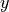given by the target variable, andgiven by its parents. The fitted model class is returned for later use.

Parameters
• all_parents (dictionary) – Dictionary of form {0:[(0, -1), (3, -2), …], 1:[], …} containing the parents estimated with PCMCI.

• selected_variables (list of integers, optional (default: range(N))) – Specify to estimate parents only for selected variables. If None is passed, parents are estimated for all variables.

• tau_max (int, optional (default: None)) – Maximum time lag. If None, the maximum lag in all_parents is used.

• cut_off ({'2xtau_max', 'max_lag', 'max_lag_or_tau_max'}) – How many samples to cutoff at the beginning. The default is ‘max_lag_or_tau_max’, which uses the maximum of tau_max and the conditions. This is useful to compare multiple models on the same sample. Other options are ‘2xtau_max’, which guarantees that MCI tests are all conducted on the same samples. For modeling, can be used, Last, ‘max_lag’ uses as much samples as possible.

• return_data (bool, optional (default: False)) – Whether to save the data array.

Returns

fit_results – Returns the sklearn model after fitting. Also returns the data transformation parameters.

Return type

dictionary of sklearn model objects for each variable

Derived classes:

class tigramite.models.LinearMediation(dataframe, model_params=None, data_transform=StandardScaler(copy=True, with_mean=True, with_std=True), mask_type=None, verbosity=0)[source]

Linear mediation analysis for time series models.

Fits linear model to parents and provides functions to return measures such as causal effect, mediated causal effect, average causal effect, etc. as described in 4.

Notes

This class implements the following causal mediation measures introduced in 4:

• causal effect (CE)

• mediated causal effect (MCE)

• average causal effect (ACE)

• average causal susceptibility (ACS)

• average mediated causal effect (AMCE)

Consider a simple model of a causal chain as given in the Example with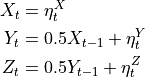Here the link coefficient of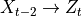is zero while the causal effect is 0.25. MCE throughis 0.25 implying that all of the the CE is explained by. ACE fromis 0.37 since it has CE 0.5 onand 0.25 on.

Examples

>>> numpy.random.seed(42)
>>> links_coeffs = {0: [], 1: [((0, -1), 0.5)], 2: [((1, -1), 0.5)]}
>>> data, true_parents = pp.var_process(links_coeffs, T=1000)
>>> dataframe = pp.DataFrame(data)
>>> med = LinearMediation(dataframe=dataframe)
>>> med.fit_model(all_parents=true_parents, tau_max=3)
>>> print "Link coefficient (0, -2) --> 2: ", med.get_coeff(i=0, tau=-2, j=2)
>>> print "Causal effect (0, -2) --> 2: ", med.get_ce(i=0, tau=-2, j=2)
>>> print "Mediated Causal effect (0, -2) --> 2 through 1: ", med.get_mce(i=0, tau=-2, j=2, k=1)
>>> print "Average Causal Effect: ", med.get_all_ace()
>>> print "Average Causal Susceptibility: ", med.get_all_acs()
>>> print "Average Mediated Causal Effect: ", med.get_all_amce()
Link coefficient (0, -2) --> 2:  0.0
Causal effect (0, -2) --> 2:  0.250648072987
Mediated Causal effect (0, -2) --> 2 through 1:  0.250648072987
Average Causal Effect:  [ 0.36897445  0.25718002  0.        ]
Average Causal Susceptibility:  [ 0.          0.24365041  0.38250406]
Average Mediated Causal Effect:  [ 0.          0.12532404  0.        ]


References

4(1,2,3)

J. Runge et al. (2015): Identifying causal gateways and mediators in complex spatio-temporal systems. Nature Communications, 6, 8502. http://doi.org/10.1038/ncomms9502

Parameters
• dataframe (data object) – Tigramite dataframe object. It must have the attributes dataframe.values yielding a numpy array of shape (observations T, variables N) and optionally a mask of the same shape and a missing values flag.

• model_params (dictionary, optional (default: None)) – Optional parameters passed on to sklearn model

• data_transform (sklearn preprocessing object, optional (default: None)) – Used to transform data prior to fitting. For example, sklearn.preprocessing.StandardScaler for simple standardization. The fitted parameters are stored.

• mask_type ({'y','x','z','xy','xz','yz','xyz'}) – Masking mode: Indicators for which variables in the dependence measure I(X; Y | Z) the samples should be masked. If None, ‘y’ is used, which excludes all time slices containing masked samples in Y. Explained in 1.

• verbosity (int, optional (default: 0)) – Level of verbosity.

fit_model(all_parents, tau_max=None)[source]

Fit linear time series model.

Fits a sklearn.linear_model.LinearRegression model to the parents of each variable and computes the coefficient matrices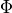and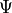as described in 4.

Parameters
• all_parents (dictionary) – Dictionary of form {0:[(0, -1), (3, -2), …], 1:[], …} containing the parents estimated with PCMCI.

• tau_max (int, optional (default: None)) – Maximum time lag. If None, the maximum lag in all_parents is used.

get_ace(i, lag_mode='absmax', exclude_i=True)[source]

Returns the average causal effect.

This is the average causal effect (ACE) emanating from variable i to any other variable. With lag_mode=’absmax’ this is based on the lag of maximum CE for each pair.

Parameters
• i (int) – Index of cause variable.

• lag_mode ({'absmax', 'all_lags'}) – Lag mode. Either average across all lags between each pair or only at the lag of maximum absolute causal effect.

• exclude_i (bool, optional (default: True)) – Whether to exclude causal effects on the variable itself at later lags.

Returns

ace – Average Causal Effect.

Return type

float

get_acs(j, lag_mode='absmax', exclude_j=True)[source]

Returns the average causal susceptibility.

This is the Average Causal Susceptibility (ACS) affecting a variable j from any other variable. With lag_mode=’absmax’ this is based on the lag of maximum CE for each pair.

Parameters
• j (int) – Index of variable.

• lag_mode ({'absmax', 'all_lags'}) – Lag mode. Either average across all lags between each pair or only at the lag of maximum absolute causal effect.

• exclude_j (bool, optional (default: True)) – Whether to exclude causal effects on the variable itself at previous lags.

Returns

acs – Average Causal Susceptibility.

Return type

float

get_all_ace(lag_mode='absmax', exclude_i=True)[source]

Returns the average causal effect for all variables.

This is the average causal effect (ACE) emanating from variable i to any other variable. With lag_mode=’absmax’ this is based on the lag of maximum CE for each pair.

Parameters
• lag_mode ({'absmax', 'all_lags'}) – Lag mode. Either average across all lags between each pair or only at the lag of maximum absolute causal effect.

• exclude_i (bool, optional (default: True)) – Whether to exclude causal effects on the variable itself at later lags.

Returns

ace – Average Causal Effect for each variable.

Return type

array of shape (N,)

get_all_acs(lag_mode='absmax', exclude_j=True)[source]

Returns the average causal susceptibility.

This is the Average Causal Susceptibility (ACS) for each variable from any other variable. With lag_mode=’absmax’ this is based on the lag of maximum CE for each pair.

Parameters
• lag_mode ({'absmax', 'all_lags'}) – Lag mode. Either average across all lags between each pair or only at the lag of maximum absolute causal effect.

• exclude_j (bool, optional (default: True)) – Whether to exclude causal effects on the variable itself at previous lags.

Returns

acs – Average Causal Susceptibility.

Return type

array of shape (N,)

get_all_amce(lag_mode='absmax', exclude_k=True, exclude_self_effects=True)[source]

Returns the average mediated causal effect.

This is the Average Mediated Causal Effect (AMCE) through all variables With lag_mode=’absmax’ this is based on the lag of maximum CE for each pair.

Parameters
• lag_mode ({'absmax', 'all_lags'}) – Lag mode. Either average across all lags between each pair or only at the lag of maximum absolute causal effect.

• exclude_k (bool, optional (default: True)) – Whether to exclude causal effects through the variable itself at previous lags.

• exclude_self_effects (bool, optional (default: True)) – Whether to exclude causal self effects of variables on themselves.

Returns

amce – Average Mediated Causal Effect.

Return type

array of shape (N,)

get_amce(k, lag_mode='absmax', exclude_k=True, exclude_self_effects=True)[source]

Returns the average mediated causal effect.

This is the Average Mediated Causal Effect (AMCE) through a variable k With lag_mode=’absmax’ this is based on the lag of maximum CE for each pair.

Parameters
• k (int) – Index of variable.

• lag_mode ({'absmax', 'all_lags'}) – Lag mode. Either average across all lags between each pair or only at the lag of maximum absolute causal effect.

• exclude_k (bool, optional (default: True)) – Whether to exclude causal effects through the variable itself at previous lags.

• exclude_self_effects (bool, optional (default: True)) – Whether to exclude causal self effects of variables on themselves.

Returns

amce – Average Mediated Causal Effect.

Return type

float

get_ce(i, tau, j)[source]

Returns the causal effect.

This is the causal effect for (i, tau) – –> j.

Parameters
• i (int) – Index of cause variable.

• tau (int) – Lag of cause variable.

• j (int) – Index of effect variable.

Returns

ce

Return type

float

get_ce_max(i, j)[source]

Returns the causal effect.

This is the maximum absolute causal effect for i –> j across all lags.

Parameters
• i (int) – Index of cause variable.

• j (int) – Index of effect variable.

Returns

ce

Return type

float

get_coeff(i, tau, j)[source]

This is the causal effect for a particular link (i, tau) –> j.

Parameters
• i (int) – Index of cause variable.

• tau (int) – Lag of cause variable.

• j (int) – Index of effect variable.

Returns

coeff

Return type

float

get_mce(i, tau, j, k)[source]

Returns the mediated causal effect.

This is the causal effect for i –> j minus the causal effect not going through k.

Parameters
• i (int) – Index of cause variable.

• tau (int) – Lag of cause variable.

• j (int) – Index of effect variable.

• k (int) – Index of mediator variable.

Returns

mce

Return type

float

get_mediation_graph_data(i, tau, j, include_neighbors=False)[source]

Returns link and node weights for mediation analysis.

Returns array with non-zero entries for links that are on causal paths betweenandat lag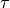. path_val_matrix contains the corresponding path coefficients and path_node_array the MCE values. tsg_path_val_matrix contains the corresponding values in the time series graph format.

Parameters
• i (int) – Index of cause variable.

• tau (int) – Lag of cause variable.

• j (int) – Index of effect variable.

• include_neighbors (bool, optional (default: False)) – Whether to include causal paths emanating from neighbors of i

Returns

graph_data – Dictionary of matrices for coloring mediation graph plots.

Return type

dictionary

get_tsg(link_matrix, val_matrix=None, include_neighbors=False)[source]

Returns time series graph matrix.

Constructs a matrix of shape (N*tau_max, N*tau_max) from link_matrix. This matrix can be used for plotting the time series graph and analyzing causal pathways.

Matrix of significant links. Must be of same shape as val_matrix. Either sig_thres or link_matrix has to be provided.

val_matrixarray_like

Matrix of shape (N, N, tau_max+1) containing test statistic values.

include_neighborsbool, optional (default: False)

Whether to include causal paths emanating from neighbors of i

Returns

tsg – Time series graph matrix.

Return type

array of shape (N*tau_max, N*tau_max)

get_val_matrix()[source]

Returns the matrix of linear coefficients.

Format is val_matrix[i, j, tau] denotes coefficient of link i –tau–> j.

Returns

val_matrix – Matrix of linear coefficients, shape (N, N, tau_max + 1).

Return type

array

net_to_tsg(row, lag, max_lag)[source]

Helper function to translate from network to time series graph.

tsg_to_net(node, max_lag)[source]

Helper function to translate from time series graph to network.

class tigramite.models.Prediction(dataframe, train_indices, test_indices, prediction_model, cond_ind_test=None, data_transform=None, verbosity=0)[source]

Prediction class for time series models.

Allows to fit and predict from any sklearn model. The optimal predictors can be estimated using PCMCI. Also takes care of missing values, masking and preprocessing.

Parameters
• dataframe (data object) – Tigramite dataframe object. It must have the attributes dataframe.values yielding a numpy array of shape (observations T, variables N) and optionally a mask of the same shape and a missing values flag.

• train_indices (array-like) – Either boolean array or time indices marking the training data.

• test_indices (array-like) – Either boolean array or time indices marking the test data.

• prediction_model (sklearn model object) – For example, sklearn.linear_model.LinearRegression() for a linear regression model.

• cond_ind_test (Conditional independence test object, optional) – Only needed if predictors are estimated with causal algorithm. The class will be initialized with masking set to the training data.

• data_transform (sklearn preprocessing object, optional (default: None)) – Used to transform data prior to fitting. For example, sklearn.preprocessing.StandardScaler for simple standardization. The fitted parameters are stored.

• verbosity (int, optional (default: 0)) – Level of verbosity.

fit(target_predictors, selected_targets=None, tau_max=None, return_data=False)[source]

Fit time series model.

Wrapper around Models.get_fit(). To each variable in selected_targets, the sklearn model is fitted withgiven by the target variable, andgiven by its predictors. The fitted model class is returned for later use.

Parameters
• target_predictors (dictionary) – Dictionary of form {0:[(0, -1), (3, -2), …], 1:[], …} containing the predictors estimated with PCMCI.

• selected_targets (list of integers, optional (default: range(N))) – Specify to fit model only for selected targets. If None is passed, models are estimated for all variables.

• tau_max (int, optional (default: None)) – Maximum time lag. If None, the maximum lag in target_predictors is used.

• return_data (bool, optional (default: False)) – Whether to save the data array.

Returns

self

Return type

instance of self

get_predictors(selected_targets=None, selected_links=None, steps_ahead=1, tau_max=1, pc_alpha=0.2, max_conds_dim=None, max_combinations=1)[source]

Estimate predictors using PC1 algorithm.

Wrapper around PCMCI.run_pc_stable that estimates causal predictors. The lead time can be specified by steps_ahead.

Parameters
• selected_targets (list of ints, optional (default: None)) – List of variables to estimate predictors of. If None, predictors of all variables are estimated.

• selected_links (dict or None) – Dictionary of form {0:[(0, -1), (3, -2), …], 1:[], …} specifying whether only selected links should be tested. If None is passed, all links are tested

• steps_ahead (int, default: 1) – Minimum time lag to test. Useful for multi-step ahead predictions.

• tau_max (int, default: 1) – Maximum time lag. Must be larger or equal to tau_min.

• pc_alpha (float or list of floats, default: 0.2) – Significance level in algorithm. If a list or None is passed, the pc_alpha level is optimized for every variable across the given pc_alpha values using the score computed in cond_ind_test.get_model_selection_criterion()

• max_conds_dim (int or None) – Maximum number of conditions to test. If None is passed, this number is unrestricted.

• max_combinations (int, default: 1) – Maximum number of combinations of conditions of current cardinality to test. Defaults to 1 for PC_1 algorithm. For original PC algorithm a larger number, such as 10, can be used.

Returns

predictors – Dictionary of form {0:[(0, -1), (3, -2), …], 1:[], …} containing estimated predictors.

Return type

dict

get_test_array()[source]

Returns test array.

get_train_array(j)[source]

Returns training array.

predict(target, new_data=None, pred_params=None, cut_off='max_lag_or_tau_max')[source]

Predict target variable with fitted model.

Uses the model.predict() function of the sklearn model.

Parameters
• target (int) – Index of target variable.

• new_data (data object, optional) – New Tigramite dataframe object with optional new mask.

• pred_params (dict, optional) – Optional parameters passed on to sklearn prediction function.

• cut_off ({'2xtau_max', 'max_lag', 'max_lag_or_tau_max'}) – How many samples to cutoff at the beginning. The default is ‘2xtau_max’, which guarantees that MCI tests are all conducted on the same samples. For modeling, ‘max_lag_or_tau_max’ can be used, which uses the maximum of tau_max and the conditions, which is useful to compare multiple models on the same sample. Last, ‘max_lag’ uses as much samples as possible.

Returns

Return type

Results from prediction.

# tigramite.plotting: Plotting functions¶

Tigramite plotting package.

tigramite.plotting.plot_graph(val_matrix, var_names=None, fig_ax=None, figsize=None, sig_thres=None, link_matrix=None, save_name=None, link_colorbar_label='MCI', node_colorbar_label='auto-MCI', link_width=None, link_attribute=None, node_pos=None, arrow_linewidth=30.0, vmin_edges=-1, vmax_edges=1.0, edge_ticks=0.4, cmap_edges='RdBu_r', vmin_nodes=0, vmax_nodes=1.0, node_ticks=0.4, cmap_nodes='OrRd', node_size=20, arrowhead_size=20, curved_radius=0.2, label_fontsize=10, alpha=1.0, node_label_size=10, link_label_fontsize=6, lag_array=None, network_lower_bound=0.2, show_colorbar=True)[source]

Creates a network plot.

This is still in beta. The network is defined either from True values in link_matrix, or from thresholding the val_matrix with sig_thres. Nodes denote variables, straight links contemporaneous dependencies and curved arrows lagged dependencies. The node color denotes the maximal absolute auto-dependency and the link color the value at the lag with maximal absolute cross-dependency. The link label lists the lags with significant dependency in order of absolute magnitude. The network can also be plotted over a map drawn before on the same axis. Then the node positions can be supplied in appropriate axis coordinates via node_pos.

Parameters
• val_matrix (array_like) – Matrix of shape (N, N, tau_max+1) containing test statistic values.

• var_names (list, optional (default: None)) – List of variable names. If None, range(N) is used.

• fig_ax (tuple of figure and axis object, optional (default: None)) – Figure and axes instance. If None they are created.

• figsize (tuple) – Size of figure.

• sig_thres (array-like, optional (default: None)) – Matrix of significance thresholds. Must be of same shape as val_matrix. Either sig_thres or link_matrix has to be provided.

• link_matrix (bool array-like, optional (default: None)) – Matrix of significant links. Must be of same shape as val_matrix. Either sig_thres or link_matrix has to be provided.

• save_name (str, optional (default: None)) – Name of figure file to save figure. If None, figure is shown in window.

• link_colorbar_label (str, optional (default: 'MCI')) – Test statistic label.

• node_colorbar_label (str, optional (default: 'auto-MCI')) – Test statistic label for auto-dependencies.

• link_width (array-like, optional (default: None)) – Array of val_matrix.shape specifying relative link width with maximum given by arrow_linewidth. If None, all links have same width.

• link_attribute (array-like, optional (default: None)) – String array of val_matrix.shape specifying link attributes.

• node_pos (dictionary, optional (default: None)) – Dictionary of node positions in axis coordinates of form node_pos = {‘x’:array of shape (N,), ‘y’:array of shape(N)}. These coordinates could have been transformed before for basemap plots.

• arrow_linewidth (float, optional (default: 30)) – Linewidth.

• vmin_edges (float, optional (default: -1)) – Link colorbar scale lower bound.

• vmax_edges (float, optional (default: 1)) – Link colorbar scale upper bound.

• edge_ticks (float, optional (default: 0.4)) – Link tick mark interval.

• cmap_edges (str, optional (default: 'RdBu_r')) – Colormap for links.

• vmin_nodes (float, optional (default: 0)) – Node colorbar scale lower bound.

• vmax_nodes (float, optional (default: 1)) – Node colorbar scale upper bound.

• node_ticks (float, optional (default: 0.4)) – Node tick mark interval.

• cmap_nodes (str, optional (default: 'OrRd')) – Colormap for links.

• node_size (int, optional (default: 20)) – Node size.

• arrowhead_size (int, optional (default: 20)) – Size of link arrow head. Passed on to FancyArrowPatch object.

• float, optional (default (curved_radius,) – Curvature of links. Passed on to FancyArrowPatch object.

• label_fontsize (int, optional (default: 10)) – Fontsize of colorbar labels.

• alpha (float, optional (default: 1.)) – Opacity.

• node_label_size (int, optional (default: 10)) – Fontsize of node labels.

• lag_array (array, optional (default: None)) – Optional specification of lags overwriting np.arange(0, tau_max+1)

• network_lower_bound (float, optional (default: 0.2)) – Fraction of vertical space below graph plot.

• show_colorbar (bool) – Whether to show colorbars for links and nodes.

tigramite.plotting.plot_lagfuncs(val_matrix, name=None, setup_args={}, add_lagfunc_args={})[source]

Wrapper helper function to plot lag functions.

Sets up the matrix object and plots the lagfunction, see parameters in setup_matrix and add_lagfuncs.

Parameters
• val_matrix (array_like) – Matrix of shape (N, N, tau_max+1) containing test statistic values.

• name (str, optional (default: None)) – File name. If None, figure is shown in window.

• setup_args (dict) – Arguments for setting up the lag function matrix, see doc of setup_matrix.

Returns

matrix – Further lag functions can be overlaid using the matrix.add_lagfuncs(val_matrix) function.

Return type

object

tigramite.plotting.plot_mediation_graph(path_val_matrix, path_node_array=None, var_names=None, fig_ax=None, figsize=None, save_name=None, link_colorbar_label='link coeff. (edge color)', node_colorbar_label='MCE (node color)', link_width=None, node_pos=None, arrow_linewidth=30.0, vmin_edges=-1, vmax_edges=1.0, edge_ticks=0.4, cmap_edges='RdBu_r', vmin_nodes=-1.0, vmax_nodes=1.0, node_ticks=0.4, cmap_nodes='RdBu_r', node_size=20, arrowhead_size=20, curved_radius=0.2, label_fontsize=10, lag_array=None, alpha=1.0, node_label_size=10, link_label_fontsize=6, network_lower_bound=0.2)[source]

Creates a network plot visualizing the pathways of a mediation analysis.

This is still in beta. The network is defined from non-zero entries in path_val_matrix. Nodes denote variables, straight links contemporaneous dependencies and curved arrows lagged dependencies. The node color denotes the mediated causal effect (MCE) and the link color the value at the lag with maximal link coefficient. The link label lists the lags with significant dependency in order of absolute magnitude. The network can also be plotted over a map drawn before on the same axis. Then the node positions can be supplied in appropriate axis coordinates via node_pos.

Parameters
• path_val_matrix (array_like) – Matrix of shape (N, N, tau_max+1) containing link weight values.

• path_node_array (array_like) – Array of shape (N,) containing node values.

• var_names (list, optional (default: None)) – List of variable names. If None, range(N) is used.

• fig_ax (tuple of figure and axis object, optional (default: None)) – Figure and axes instance. If None they are created.

• figsize (tuple) – Size of figure.

• save_name (str, optional (default: None)) – Name of figure file to save figure. If None, figure is shown in window.

• node_colorbar_label (str, optional (default: 'MCE (node color)')) – Node colorbar label.

• link_width (array-like, optional (default: None)) – Array of val_matrix.shape specifying relative link width with maximum given by arrow_linewidth. If None, all links have same width.

• node_pos (dictionary, optional (default: None)) – Dictionary of node positions in axis coordinates of form node_pos = {‘x’:array of shape (N,), ‘y’:array of shape(N)}. These coordinates could have been transformed before for basemap plots.

• arrow_linewidth (float, optional (default: 30)) – Linewidth.

• vmin_edges (float, optional (default: -1)) – Link colorbar scale lower bound.

• vmax_edges (float, optional (default: 1)) – Link colorbar scale upper bound.

• edge_ticks (float, optional (default: 0.4)) – Link tick mark interval.

• cmap_edges (str, optional (default: 'RdBu_r')) – Colormap for links.

• vmin_nodes (float, optional (default: 0)) – Node colorbar scale lower bound.

• vmax_nodes (float, optional (default: 1)) – Node colorbar scale upper bound.

• node_ticks (float, optional (default: 0.4)) – Node tick mark interval.

• cmap_nodes (str, optional (default: 'OrRd')) – Colormap for links.

• node_size (int, optional (default: 20)) – Node size.

• arrowhead_size (int, optional (default: 20)) – Size of link arrow head. Passed on to FancyArrowPatch object.

• float, optional (default (curved_radius,) – Curvature of links. Passed on to FancyArrowPatch object.

• label_fontsize (int, optional (default: 10)) – Fontsize of colorbar labels.

• alpha (float, optional (default: 1.)) – Opacity.

• node_label_size (int, optional (default: 10)) – Fontsize of node labels.

• network_lower_bound (float, optional (default: 0.2)) – Fraction of vertical space below graph plot.

• lag_array (array, optional (default: None)) – Optional specification of lags overwriting np.arange(0, tau_max+1)

tigramite.plotting.plot_mediation_time_series_graph(path_node_array, tsg_path_val_matrix, var_names=None, fig_ax=None, figsize=None, link_colorbar_label='link coeff. (edge color)', node_colorbar_label='MCE (node color)', save_name=None, link_width=None, arrow_linewidth=20.0, vmin_edges=-1, vmax_edges=1.0, edge_ticks=0.4, cmap_edges='RdBu_r', order=None, vmin_nodes=-1.0, vmax_nodes=1.0, node_ticks=0.4, cmap_nodes='RdBu_r', node_size=10, arrowhead_size=20, curved_radius=0.2, label_fontsize=10, alpha=1.0, node_label_size=10, label_space_left=0.1, label_space_top=0.0, network_lower_bound=0.2)[source]

Creates a mediation time series graph plot.

This is still in beta. The time series graph’s links are colored by val_matrix.

Parameters
• tsg_path_val_matrix (array_like) – Matrix of shape (N*tau_max, N*tau_max) containing link weight values.

• path_node_array (array_like) – Array of shape (N,) containing node values.

• var_names (list, optional (default: None)) – List of variable names. If None, range(N) is used.

• fig_ax (tuple of figure and axis object, optional (default: None)) – Figure and axes instance. If None they are created.

• figsize (tuple) – Size of figure.

• save_name (str, optional (default: None)) – Name of figure file to save figure. If None, figure is shown in window.

• node_colorbar_label (str, optional (default: 'MCE (node color)')) – Node colorbar label.

• link_width (array-like, optional (default: None)) – Array of val_matrix.shape specifying relative link width with maximum given by arrow_linewidth. If None, all links have same width.

• order (list, optional (default: None)) – order of variables from top to bottom.

• arrow_linewidth (float, optional (default: 30)) – Linewidth.

• vmin_edges (float, optional (default: -1)) – Link colorbar scale lower bound.

• vmax_edges (float, optional (default: 1)) – Link colorbar scale upper bound.

• edge_ticks (float, optional (default: 0.4)) – Link tick mark interval.

• cmap_edges (str, optional (default: 'RdBu_r')) – Colormap for links.

• vmin_nodes (float, optional (default: 0)) – Node colorbar scale lower bound.

• vmax_nodes (float, optional (default: 1)) – Node colorbar scale upper bound.

• node_ticks (float, optional (default: 0.4)) – Node tick mark interval.

• cmap_nodes (str, optional (default: 'OrRd')) – Colormap for links.

• node_size (int, optional (default: 20)) – Node size.

• arrowhead_size (int, optional (default: 20)) – Size of link arrow head. Passed on to FancyArrowPatch object.

• float, optional (default (curved_radius,) – Curvature of links. Passed on to FancyArrowPatch object.

• label_fontsize (int, optional (default: 10)) – Fontsize of colorbar labels.

• alpha (float, optional (default: 1.)) – Opacity.

• node_label_size (int, optional (default: 10)) – Fontsize of node labels.

• label_space_left (float, optional (default: 0.1)) – Fraction of horizontal figure space to allocate left of plot for labels.

• label_space_top (float, optional (default: 0.)) – Fraction of vertical figure space to allocate top of plot for labels.

• network_lower_bound (float, optional (default: 0.2)) – Fraction of vertical space below graph plot.

tigramite.plotting.plot_time_series_graph(val_matrix, var_names=None, fig_ax=None, figsize=None, sig_thres=None, link_matrix=None, link_colorbar_label='MCI', save_name=None, link_width=None, arrow_linewidth=20.0, vmin_edges=-1, vmax_edges=1.0, edge_ticks=0.4, cmap_edges='RdBu_r', order=None, node_size=10, arrowhead_size=20, curved_radius=0.2, label_fontsize=10, alpha=1.0, node_label_size=10, label_space_left=0.1, label_space_top=0.0, network_lower_bound=0.2, undirected_style='dashed')[source]

Creates a time series graph.

This is still in beta. The time series graph’s links are colored by val_matrix.

Parameters
• val_matrix (array_like) – Matrix of shape (N, N, tau_max+1) containing test statistic values.

• var_names (list, optional (default: None)) – List of variable names. If None, range(N) is used.

• fig_ax (tuple of figure and axis object, optional (default: None)) – Figure and axes instance. If None they are created.

• figsize (tuple) – Size of figure.

• sig_thres (array-like, optional (default: None)) – Matrix of significance thresholds. Must be of same shape as val_matrix. Either sig_thres or link_matrix has to be provided.

• link_matrix (bool array-like, optional (default: None)) – Matrix of significant links. Must be of same shape as val_matrix. Either sig_thres or link_matrix has to be provided.

• save_name (str, optional (default: None)) – Name of figure file to save figure. If None, figure is shown in window.

• link_colorbar_label (str, optional (default: 'MCI')) – Test statistic label.

• link_width (array-like, optional (default: None)) – Array of val_matrix.shape specifying relative link width with maximum given by arrow_linewidth. If None, all links have same width.

• order (list, optional (default: None)) –

• of variables from top to bottom. (order) –

• arrow_linewidth (float, optional (default: 30)) – Linewidth.

• vmin_edges (float, optional (default: -1)) – Link colorbar scale lower bound.

• vmax_edges (float, optional (default: 1)) – Link colorbar scale upper bound.

• edge_ticks (float, optional (default: 0.4)) – Link tick mark interval.

• cmap_edges (str, optional (default: 'RdBu_r')) – Colormap for links.

• node_size (int, optional (default: 20)) – Node size.

• arrowhead_size (int, optional (default: 20)) – Size of link arrow head. Passed on to FancyArrowPatch object.

• float, optional (default (curved_radius,) – Curvature of links. Passed on to FancyArrowPatch object.

• label_fontsize (int, optional (default: 10)) – Fontsize of colorbar labels.

• alpha (float, optional (default: 1.)) – Opacity.

• node_label_size (int, optional (default: 10)) – Fontsize of node labels.

• label_space_left (float, optional (default: 0.1)) – Fraction of horizontal figure space to allocate left of plot for labels.

• label_space_top (float, optional (default: 0.)) – Fraction of vertical figure space to allocate top of plot for labels.

• network_lower_bound (float, optional (default: 0.2)) – Fraction of vertical space below graph plot.

• undirected_style (string, optional (default: 'dashed')) – Style of undirected contemporaneous links.

tigramite.plotting.plot_timeseries(dataframe=None, save_name=None, fig_axes=None, figsize=None, var_units=None, time_label='time', use_mask=False, grey_masked_samples=False, data_linewidth=1.0, skip_ticks_data_x=1, skip_ticks_data_y=2, label_fontsize=8)[source]

Create and save figure of stacked panels with time series.

Parameters
• dataframe (data object, optional) – This is the Tigramite dataframe object. It has the attributes dataframe.values yielding a np array of shape (observations T, variables N) and optionally a mask of the same shape.

• save_name (str, optional (default: None)) – Name of figure file to save figure. If None, figure is shown in window.

• fig_axes (subplots instance, optional (default: None)) – Figure and axes instance. If None they are created as fig, axes = pyplot.subplots(N,…)

• figsize (tuple of floats, optional (default: None)) – Figure size if new figure is created. If None, default pyplot figsize is used.

• var_units (list of str, optional (default: None)) – Units of variables.

• time_label (str, optional (default: '')) – Label of time axis.

• use_mask (bool, optional (default: False)) – Whether to use masked data.

• grey_masked_samples (bool, optional (default: False)) – Whether to mark masked samples by grey fills (‘fill’) or grey data (‘data’).

• data_linewidth (float, optional (default: 1.)) – Linewidth.

• skip_ticks_data_x (int, optional (default: 1)) – Skip every other tickmark.

• skip_ticks_data_y (int, optional (default: 2)) – Skip every other tickmark.

• label_fontsize (int, optional (default: 10)) – Fontsize of variable labels.

class tigramite.plotting.setup_matrix(N, tau_max, var_names=None, figsize=None, minimum=-1, maximum=1, label_space_left=0.1, label_space_top=0.05, legend_width=0.15, legend_fontsize=10, x_base=1.0, y_base=0.5, plot_gridlines=False, lag_units='', lag_array=None, label_fontsize=10)[source]

Create matrix of lag function panels.

Class to setup figure object. The function add_lagfuncs(…) allows to plot the val_matrix of shape (N, N, tau_max+1). Multiple lagfunctions can be overlaid for comparison.

Parameters
• N (int) – Number of variables

• tau_max (int) – Maximum time lag.

• var_names (list, optional (default: None)) – List of variable names. If None, range(N) is used.

• figsize (tuple of floats, optional (default: None)) – Figure size if new figure is created. If None, default pyplot figsize is used.

• minimum (int, optional (default: -1)) – Lower y-axis limit.

• maximum (int, optional (default: 1)) – Upper y-axis limit.

• label_space_left (float, optional (default: 0.1)) – Fraction of horizontal figure space to allocate left of plot for labels.

• label_space_top (float, optional (default: 0.05)) – Fraction of vertical figure space to allocate top of plot for labels.

• legend_width (float, optional (default: 0.15)) – Fraction of horizontal figure space to allocate right of plot for legend.

• x_base (float, optional (default: 1.)) – x-tick intervals to show.

• y_base (float, optional (default: .4)) – y-tick intervals to show.

• plot_gridlines (bool, optional (default: False)) – Whether to show a grid.

• lag_units (str, optional (default: '')) –

• lag_array (array, optional (default: None)) – Optional specification of lags overwriting np.arange(0, tau_max+1)

• label_fontsize (int, optional (default: 10)) – Fontsize of variable labels.

add_lagfuncs(val_matrix, sig_thres=None, conf_matrix=None, color='black', label=None, two_sided_thres=True, marker='.', markersize=5, alpha=1.0)[source]

Add lag function plot from val_matrix array.

Parameters
• val_matrix (array_like) – Matrix of shape (N, N, tau_max+1) containing test statistic values.

• sig_thres (array-like, optional (default: None)) – Matrix of significance thresholds. Must be of same shape as val_matrix.

• conf_matrix (array-like, optional (default: None)) – Matrix of shape (, N, tau_max+1, 2) containing confidence bounds.

• color (str, optional (default: 'black')) – Line color.

• label (str) – Test statistic label.

• two_sided_thres (bool, optional (default: True)) – Whether to draw sig_thres for pos. and neg. values.

• marker (matplotlib marker symbol, optional (default: '.')) – Marker.

• markersize (int, optional (default: 5)) – Marker size.

• alpha (float, optional (default: 1.)) – Opacity.

savefig(name=None)[source]

Save matrix figure.

Parameters

name (str, optional (default: None)) – File name. If None, figure is shown in window.